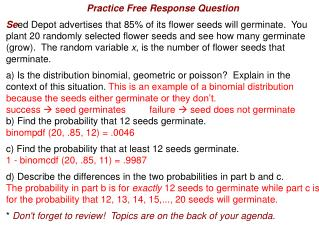DownloadDownload PresentationPractice Free Response Question

# Practice Free Response Question

Télécharger la présentation## Practice Free Response Question

- - - - - - - - - - - - - - - - - - - - - - - - - - - E N D - - - - - - - - - - - - - - - - - - - - - - - - - - -
##### Presentation Transcript

1. Practice Free Response Question Seed Depot advertises that 85% of its flower seeds will germinate. You plant 20 randomly selected flower seeds and see how many germinate (grow). The random variable x, is the number of flower seeds that germinate. a) Is the distribution binomial, geometric or poisson? Explain in the context of this situation. This is an example of a binomial distribution because the seeds either germinate or they don’t. success  seed germinates failure  seed does not germinate b) Find the probability that 12 seeds germinate. binompdf (20, .85, 12) = .0046 c) Find the probability that at least 12 seeds germinate. 1 - binomcdf (20, .85, 11) = .9987 d) Describe the differences in the two probabilities in part b and c. The probability in part b is for exactly 12 seeds to germinate while part c is for the probability that 12, 13, 14, 15,..., 20 seeds will germinate. * Don't forget to review! Topics are on the back of your agenda.

2. Attachments